# High School Physics : Understanding the Electromagnetic Spectrum

## Example Questions

### Example Question #1 : Light And Electromagnetic Waves

A microwave has a wavelength of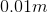. What is the speed of the wave?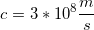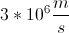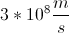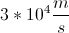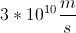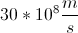Explanation:

Since a microwave is a part of the electromagnetic spectrum, its velocity will be equal to the speed of light: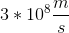Any wave on the electromagnetic spectrum will have the same velocity, though their wavelengths and frequencies will vary, defining their individual characteristics.

### Example Question #2 : Light And Electromagnetic Waves

A microwave has a frequency of. A gamma ray has a frequency of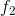. Which one has a greater speed?

The gamma ray

We need to know the wavelengths to be able to solve

We need to know the magnitude of the frequencies to solve

They have the same speed

The microwave

They have the same speed

Explanation:

Both of these waves are on the electromagnetic spectrum. Any wave on the spectrum will have the same speed, the speed of light.Radio waves, microwaves, infrared waves, visible light, ultraviolet light, and gamma rays are all on the spectrum. They all have the same ultimate speed, but vary in their wavelengths, frequencies, and energy levels.

### Example Question #3 : Light And Electromagnetic Waves

The frequency of a particular photon of infrared light is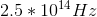. What is the wavelength of the photon?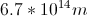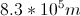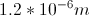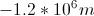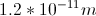Explanation:

The relationship between wavelength, frequency, and velocity is: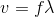In this case, because infrared light is a part of the electromagnetic spectrum, we would usefor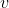.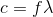We are given the speed of light and the frequency of the photon. Using these values, we can solve for the wavelength.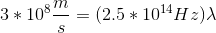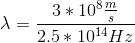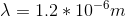### Example Question #4 : Light And Electromagnetic Waves

An electromagnetic wave travels with a frequency of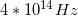. What is the wavelength of the wave?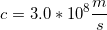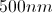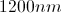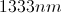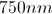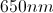Explanation:

The relationship between velocity, frequency, and wavelength of a waveform is given by the formula: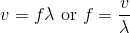In this case, we are dealing with an electromagnetic wave. All electromagnetic waves travel at the same velocity: the speed of light.

Using this value and the given frequency of the wave, we can solve for the wavelength.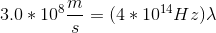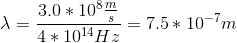Wavelength is traditionally reported in nanometers, corresponding to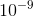.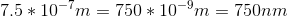### Example Question #5 : Light And Electromagnetic Waves

Radio waves and X-rays are both on the electromagnetic spectrum. Which of the following statements is true?

Both waves have the same period

Both waves travel at the same speed

None of these are true

Both waves have the same wavelength

Both waves have the same frequency